# Catch up on the latest AI articles# What Is Prompt Tuning To Optimize Prompts For High Performance?

3 main points
✔️ Proposed Prompt Tuning to learn only the Prompt portion of a frozen generic LLM
✔️ Hit accuracy close to Fine-tuning
✔️ Allows significant parameter reduction

written by Brian LesterRami Al-RfouNoah Constant
(Submitted on 18 Apr 2021 (v1), last revised 2 Sep 2021 (this version, v2))

Subjects: Computation and Language (cs.CL)code：The images used in this article are from the paper, the introductory slides, or were created based on them.

## Introduction

This article introduces research on Prompt Tuning, one of LLM's prompting techniques.

Recent high-precision LLMs such as GPT-4 and T5 have parameter counts in excess of 10 billion. Such enormity makes it possible to solve a wide variety of tasks in a generic manner.

Accordingly, efforts to use such huge LLMs to specialize in tasks in specific fields are flourishing. For example, LLMs specializing in the medical field and LLMs specializing in the educational field. In order to specialize in such fields, the mainstream method is to train additional data specific to each field on pre-trained LLMs.

This method is called additive learning and is typified by the following techniques

• Model Tuning (Fine-tuning)
• Prompt Design (Prompt Enginnering)

It is true that the issue of "how to perform additional learning at low cost" has often been discussed. This is because, as expected, learning LLMs with hundreds of billions of parameters is computationally expensive, making it impossible to do so unless one has the computing resources of a large company.

To solve such computational cost issues, the "Prompt Tuning" method was proposed in this paper.

### What is Model Tuning (Fine-tuning)?

Model Tuning is a method of updating the entire model or some of its parameters by adding a task-specific layer to the final layer of the pre-trained model in order to make the LLM specialized for each task. In this process, a large amount of data is required for the task to be solved, which must be trained into the pre-trained model.

In this way, a generic LLM can be specialized for each task and accuracy in that area can be increased.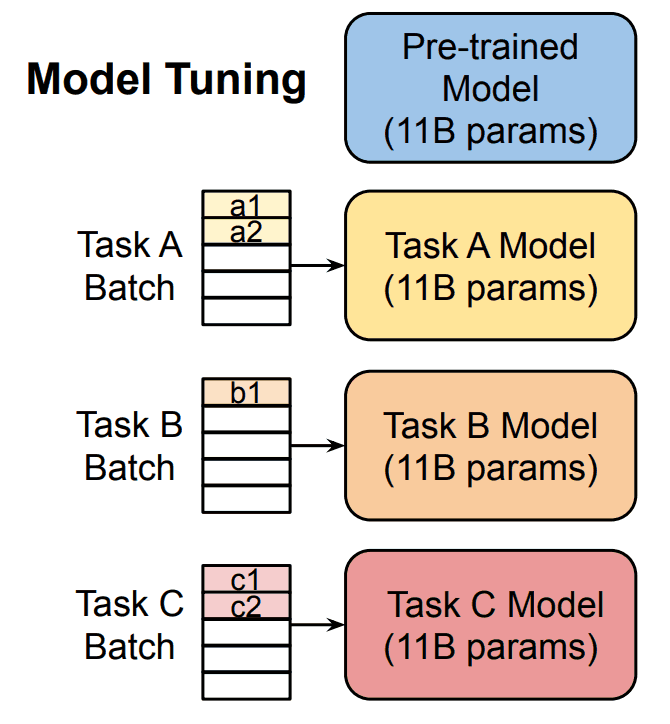### Problems with Model Tuning (Fine-tuning)

However, as mentioned earlier, training an LLM with hundreds of billions of parameters requires enormous computational resources. On top of that, creating several LLMs specialized for each task is not realistic from a resource perspective.

### What is Prompt Design (Prompt Enginnering)?

Since ChatGPT has been discussed, the term Prompt has also been used. In a nutshell, a Prompt is "a directive given to an LLM", and there are two main types of Prompts

• hard prompt
• soft prompt

Along with the term Prompt, the term Prompt Design (Prompt Enginnering) is also often used. This is a method in which the Prompt is properly designed by humans to increase accuracy without additional training of the LLM.

Various techniques have been invented for this technique, such as Few-shot and CoT, per the hard prompt.

### Problems with Prompt Design (Prompt Enginnering)

This Prompt Design requires relatively low cost. However, the authors of this study point out the following problems with these hard prompts

• Prompt designer's skill required.
• Much less accurate than Model Tuning

In particular, the following chart provides evidence for the low accuracy.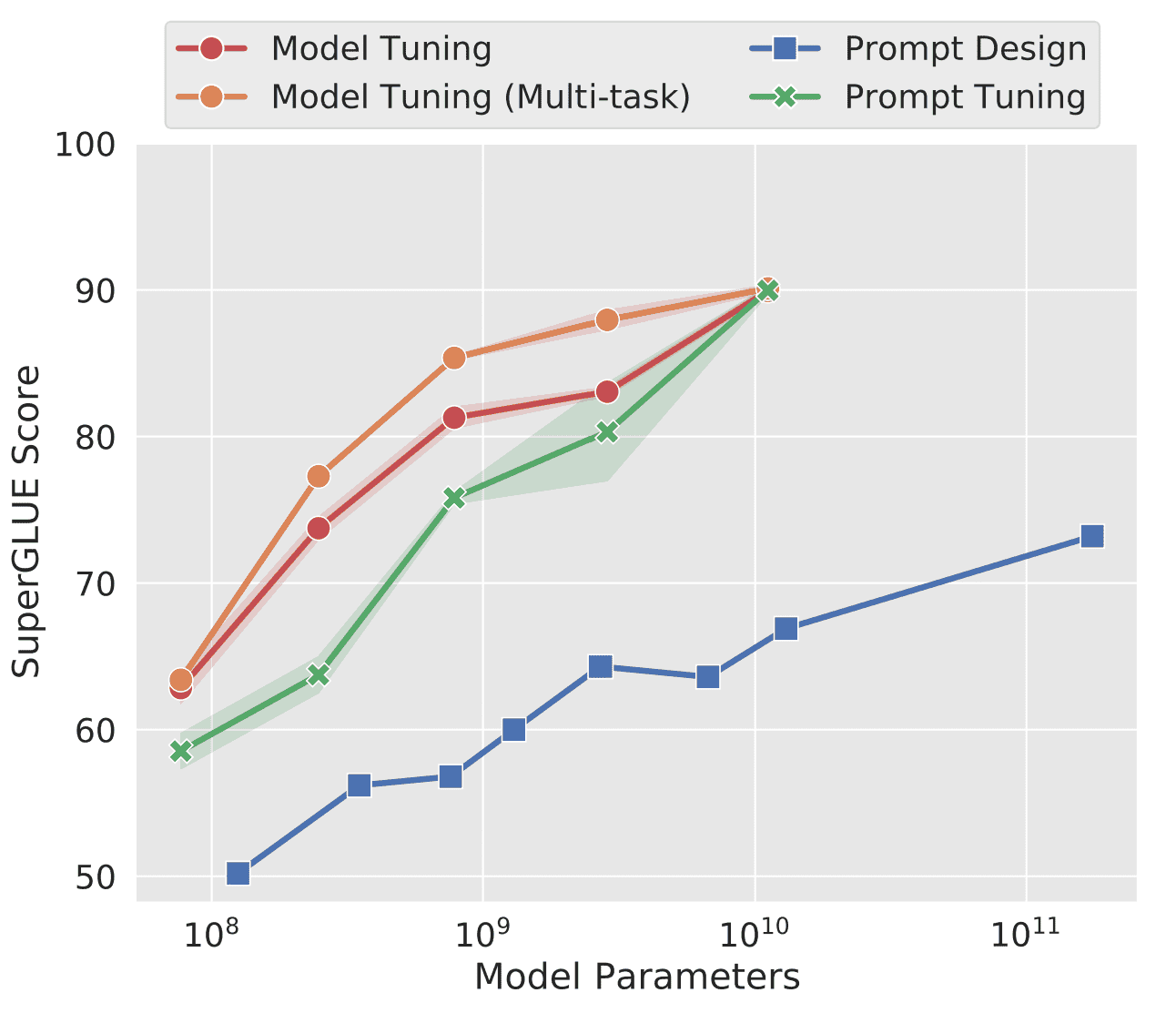Here, the vertical axis in this figure refers to SuperGLUE, "the overall score for various NLP task accuracy. The horizontal axis is the number of parameters, and as this value increases, SuperGLUE naturally increases.

It is clear from this figure that Prompt Design is the least accurate, up to 25 points or more ahead of Model Tuning.

## What is "Prompt Tuning", the method proposed in this paper?

Finally, I would like to introduce the method proposed in this paper: the Prompt Tuning method, which is to let the Prompt learn.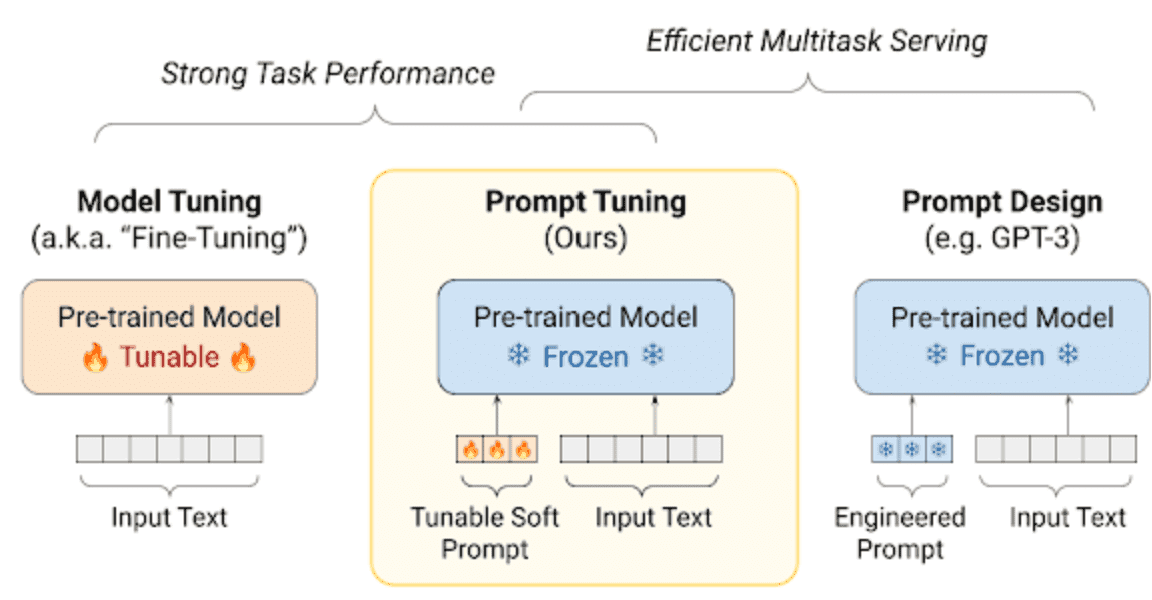From the figure above, you can see that a parameter-adjustable Prompt (Soft Prompt) is added to the input text that corresponds to the Prompt. This is given as the training vector. Note that it is also called Soft Prompt.

In Model Tuning, there are no such Soft Prompts, only input text. Also, with Prompt Design, both the Soft Prompt and the pre-trained model are frozen and not trained.

Furthermore, with Prompt Tuning, only one pre-trained model can be specialized for multiple tasks, rather than having to build a model for each task, as is the case with Model Tuning.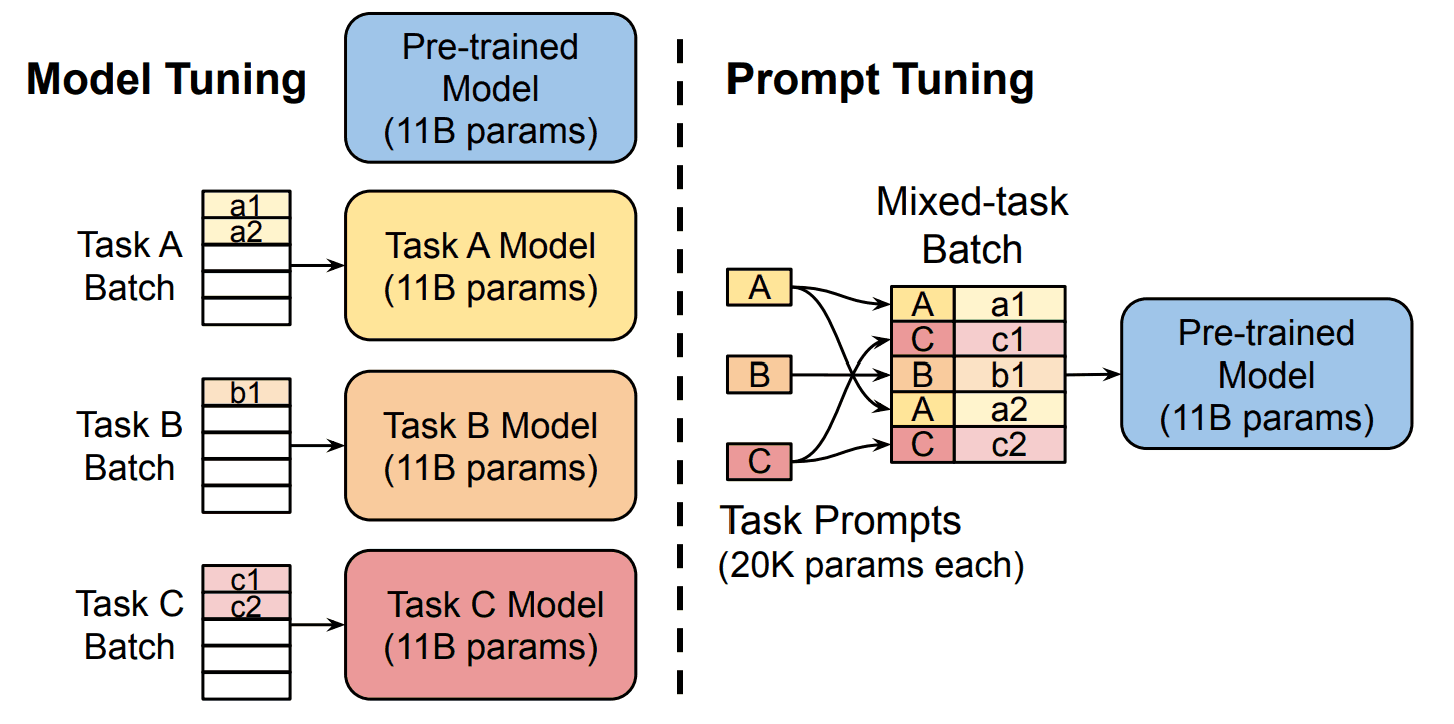Here, Soft Prompt consists of "Token Count" and "Embedding". The definitions of each are as follows

• Number of tokens: like number of words to be entered
• Embedding: vector representation of each token (embedding)

If the number of tokens in Soft Prompt is p and the vector dimension of the embedding of each token is e, then the number of parameters in Soft Promt is represented by p*e. Let us now compare the number of training parameters between Prompt Tuning and Model Tuning.

For example, if the number of tokens p in Soft Prompt is 100 and the dimensionality of the embedded representation of a word e is 4096 dimensions, the number of training parameters is as follows

• Prompt Tuning: 100*4096=409600 parameters
• Model Tuning: 11 billion parameters at most when pre-trained model is set to T5

Compared to Model Tuning, you will find that Prompt Tuning is much more efficient.

### What is the optimal Soft Prompt token count p?

The fewer the number of tokens p, or length, in Soft Prompt, the fewer the training parameters, the more efficient it will be. However, in general, the smaller the number of parameters, the lower the accuracy, but what about Prompt Tuning?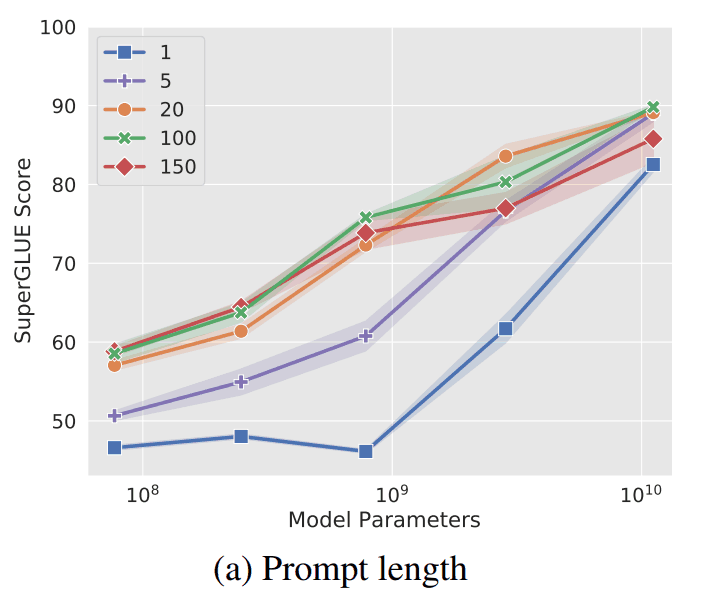In the above figure, it is obvious that the higher the number of tokens, the higher the accuracy. However, when the number of parameters is 1010 = 10 billion (comparable to the number of parameters in T5), it is clear that high accuracy is achieved even with a token count of 1.

Therefore, if a huge model is used for pre-trained models, this number of tokens will not matter much.

## Result

Although there are various validations in this study, we will focus on the following three.

• Comparison of accuracy between each method and Prompt Tuning
• Prompt Ensembling

Let's look at them in turn.

### Comparison of accuracy between each method and Prompt Tuning

In this study, comparative experiments with Model Tuning and Prompt Design are conducted to verify the accuracy of Prompt Tuning. Here, T5 is used as the pre-trained model for Model Tuning and Prompt Tuning, while GPT-3 is used for Prompt Design.

The results are shown in the following figure. This is a restatement.As can be seen, Prompt Tuning is much more powerful than Prompt Design. In addition to that, as the number of parameters increases to 1010=10 billion, Prompt Tuning reaches almost the same accuracy as Model Tuning.

The domain shift problem is the phenomenon of LLM overfitting to a data set. For example, a model trained on one dataset is not necessarily adaptable to the other dataset.

In order to verify the ability to adapt to domain shifts without such overfitting, this study will train and test separately on the QQP and MRPC data sets. For example, if we train on QQP, we will test on MRPC.

In addition, this validation includes an accuracy comparison between Model Tuning and Prompt Tuning. The results are as follows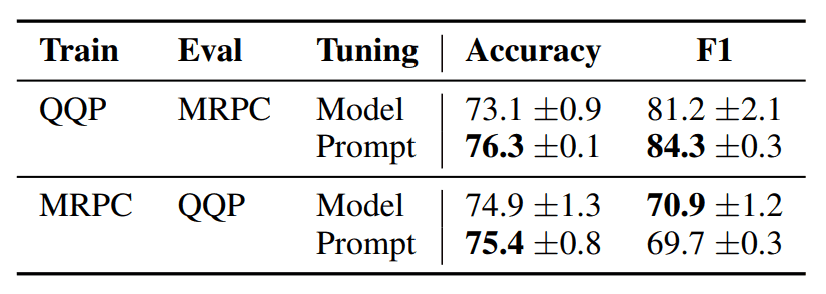In the case of QQP to MRPC, Prompt Tuning is higher for both Accuracy and F1, and for MRPC to QQP, Prompt Tuning is also higher for Accuracy.

Therefore, Prompt Tuning may be more adaptive with respect to the domain shift issue.

### Prompt Ensembling

Ensemble learning, in which the final output is calculated from the outputs of multiple models, e.g., by majority voting.

In this study, this Ensemble learning is also validated by applying it to the Prompt. Specifically, instead of preparing multiple models, multiple Prompt patterns are prepared and Ensemble learning is applied to them.

The results are as follows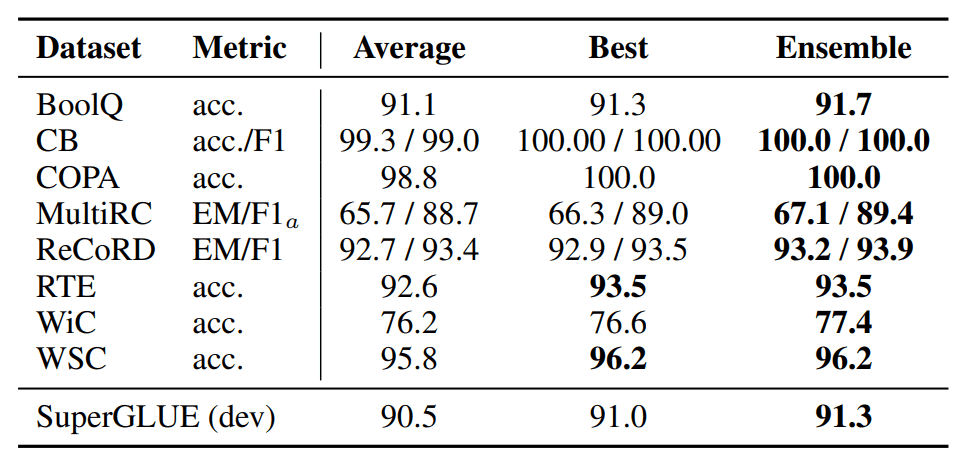The above results show that even with Prompt Tuning, high accuracy can be achieved by Ensemble learning.

## Summary

In this study, "Prompt Tuning" was proposed with the motivation of "let's optimize Prompt too".

To date, the most common method is prompt engineering (manual), in which the optimal prompt sentence is designed. However, since it is inefficient for humans to work hard on the subsequent use of LLMs after they have been trained using large amounts of data and computational resources, it has often been suggested that it is better to have LLMs automatically create the prompts as well.

I believe this study has provided a valid approach and rationale for such opinions.

In addition, this study itself is not that new, so it is expected that more effective approaches will emerge in the future. Then, all we humans have to do is to "intuitively command the LLM without worrying about prompting techniques," and the LLM may be able to take care of the later task of "improving the accuracy of the LLM" on its own.

In fact, there are agents and other related technologies out there that you should check out.

If you have any suggestions for improvement of the content of the article,